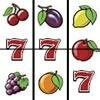Computable Minds - www.computableminds.com
109

# How a machine learns to harness a compulsive gambler?

Posted on: Dec, 8th 2012There are many people who believe that a machine isn't capable of generate random results and with this idea spend their savings betting in Internet on all kind of gambling waiting to find a pattern. In fact, the machine not only can generate random results, but also adjust them for that the prizes won are the needed for keep hooked the gambler and maximize the benefits of the casino.

The humans are very bad giving random numbers, if someone ask us that we say a number between 1 and 10 we will say more frequently the 3 or the 7 and although a lot of believe that a computer can't generate random numbers, paradoxically it can do it better than any human using mathematical functions like this:

f(x)=(a*f(x-1)+c) mod m, where f(0) is a value called seed that can be any and a,c and m are constant values of natural numbers selected as it would be appropriate.

This generator will get equiprobable random numbers between 0 and m-1 that begin to repeat as maximum getting m numbers. If m is sufficiently big, never will perceive that there are a pattern that it repeats. The majority of times a generator of this kind, or a modified version, is sufficient to make believe to anyone that the numbers are really random, although really aren't because are generated by a mathematical function, that is the reason because this kind of generators are called pseudorandom. Before one of these pseudorandom number generators could be used in an application, is necessary made a statistical analysis of the numbers that generates, to see how difficult is predict the next number. If the algorithm is good enough, this can be extremely complicated, even for a mathematic that has the help of a supercomputer.

If to a pseudorandom number generator we apply modifications adding external factors really random, such as the hour in the moment of generate the number, the seconds passed since the user began to play, the last key pressed, the mouse position, the temperature of the processor or other variables of the application, never will get a pattern that it repeats and so we will have a real random number generator.

Now that we know how a machine can generate random numbers, we will see how can be controlled that randomness. Suppose that we want that a game ends winning the user the 20% of the times and the remaining 80% will lose, because analyzing players data, we have discovered that these are the ideal values for that they bet the maximum possible, winning the minimum possible. This can be implemented simply generating random numbers between 1 and 10. If the result is less or iqual to 2, the machine will show a winner combination, if is not, will show a loser combination. Take into account that the consequence of this, will not be that when we play 100 times, 20 times will win and 80 will lose, because the results still remain random. The thing that will really happen, is that if we play infinite times, we will win the 20% of them and we will lose the 80%.

In a gaming, the benefit that we get is calculated multiplying the probability of win by the value of the prize. In all the gaming this is always much less than the cost of playing, is that to say, we will lose money. If we adjust the probabilities we can establish how much we will win the casino banking and how much will lose the gambler. In the games where the randomness isn't manipulated, as for instance the lottery, if we do these calculations we can see easily how much money we will lose with each ticket played, that always will be nearly the full price of the ticket. But for example, in a slot machine, online or not, where apparently almost all combinations have a prize, if we do the previous calculation, supposing an equiprobable distribution of results, we will get that we will win more money than the bet due to the high probabilities of win. Even without doing any calculation, intuitively will seem that this is true. The trick of the swindle is in that the slot machine would be have been programmed in the way that I've explained before, or with some technique more complex, like for instance with a hidden Markov model, with the intention of manipulate the probability of the results, causing that the combinations that give a prize will be less probable and, so that we follow giving money to the casino.

The slot machines online also have an important advantage respect to the real slot machines and is that these can identify the user and adjust the probabilities of win or lose individually for each one, in function of the quantity that they bet in each session. In this way, depending of the grade of pathological gambling, the machine will give them more or less prizes for keep them hooked.

Now you are already forewarned, the one that offers an online gambling, knows in advance how much money will get from you if you play, so be smart and don't do it.

Comments (0): Comment
Categories: ,

Share:

Copy and paste in your page:

Share
How about you!? Don't give your opinion?

Replying to the next comments:

To check if you are human answer the question correctly:

None of these data will be stored.

(Write the e-mail)

Required field.

(Write the e-mail or several e-mails separated by coma)

Required field.

To check if you are human answer the question correctly: